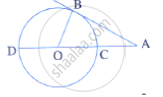Share

In the Given Figure, O is the Centre of the Circle and Ab is a Tangent at B. If Ab = 15 Cm and Ac = 7.5 Cm, Calculate the Radius of the Circle. - ICSE Class 10 - Mathematics

ConceptTangent Properties - If a Line Touches a Circle and from the Point of Contact, a Chord is Drawn, the Angles Between the Tangent and the Chord Are Respectively Equal to the Angles in the Corresponding Alternate Segments

Question

In the given figure, O is the centre of the circle and AB is a tangent at B. If AB = 15 cm and AC = 7.5 cm, calculate the radius of the circle.Solution

AB = 15 cm, AC = 7.5 cm
Let 'r' be the radius of the circle.
∴ OC = OB = r
AO = AC + OC = 7.5 + r
In Δ AOB,
AO^2 = AB^2 =OB^2

(7.5 + r)^2=15^2 +r^2

⇒ ((15+2r)/2)^2 =225 +r^2

⇒ 225 + 4r^2 +60r=900 +4r^2

⇒60r=675

⇒r=11.25cm

Therefore, r = 11.25 cm

Is there an error in this question or solution?

Video TutorialsVIEW ALL 

Solution In the Given Figure, O is the Centre of the Circle and Ab is a Tangent at B. If Ab = 15 Cm and Ac = 7.5 Cm, Calculate the Radius of the Circle. Concept: Tangent Properties - If a Line Touches a Circle and from the Point of Contact, a Chord is Drawn, the Angles Between the Tangent and the Chord Are Respectively Equal to the Angles in the Corresponding Alternate Segments.
S